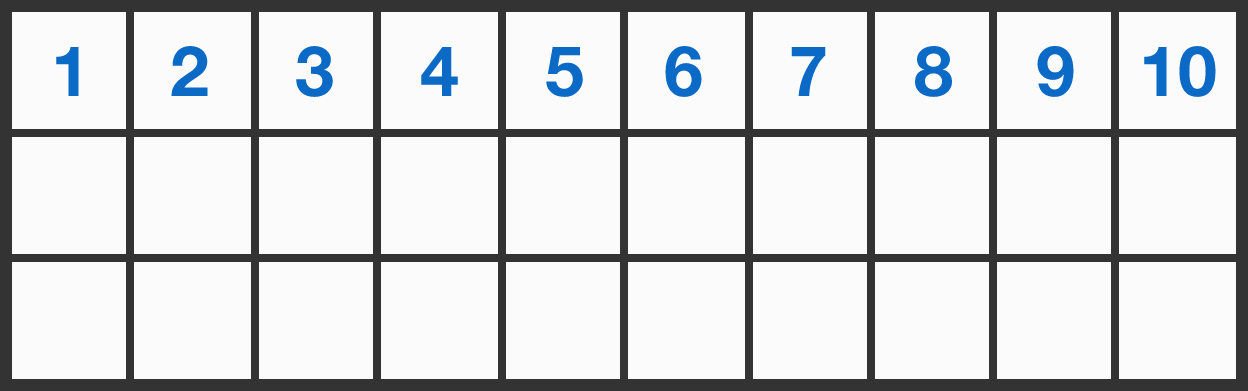# Distribute numbers in a table

Probability Level 1The $3\times 10$ grid above is to be filled with integers in the following way:

• The second row is completed with the numbers from 1 to 10 in any order.
• Each number in the third row is the sum of the two numbers above it.

Is there any way to complete the second row so that the units digits of the numbers in the third row are all different?

×

Problem Loading...

Note Loading...

Set Loading...